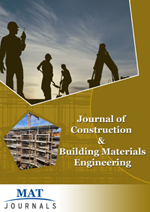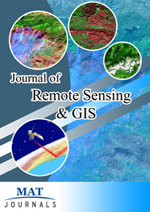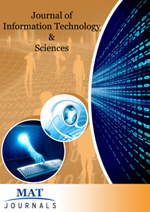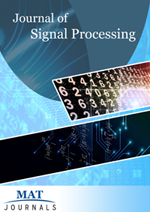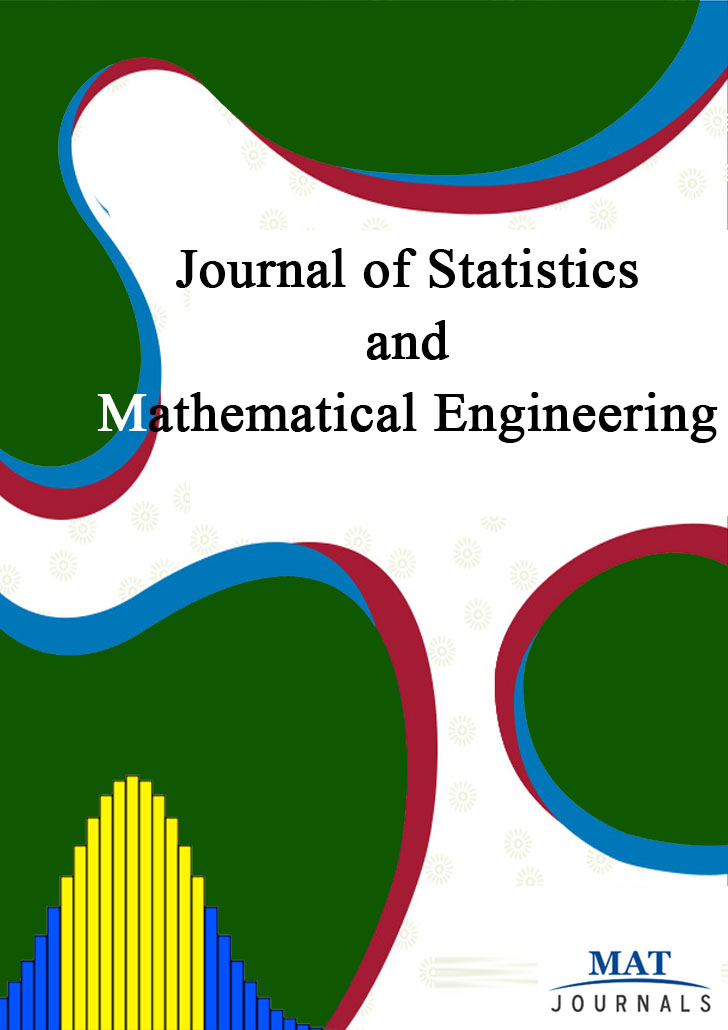### Journal of Statistics and Mathematical Engineering

Journal of Statistics and Mathematical Engineering :- is a print e-journal focused towards the rapid Publication of fundamental research papers on all areas of statistics and mathematical engineering.

Mathematical statistics is the application of mathematics to statistics, which was originally conceived as the science of the state the collection and analysis of facts about a country: its economy, land, military, population, and so forth.

• Descriptive statistics.
• Inferential statistics.
• Natural experiments.
• Observational studies.
• Statistical theorists.
• Probability distributions.
• Decision theory.
• Algebra.
• Combinatorics.
• This Journal involves the comprehensive coverage of all the aspects of statistics and mathematical engineering.